# Predicting the Life Expectancy of a Different Countries — Multiple Linear RegressionThis image was taken from retireneenew.org

# Exploratory Data Analysis (EDA)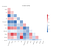Fig. 1. Correlation heat map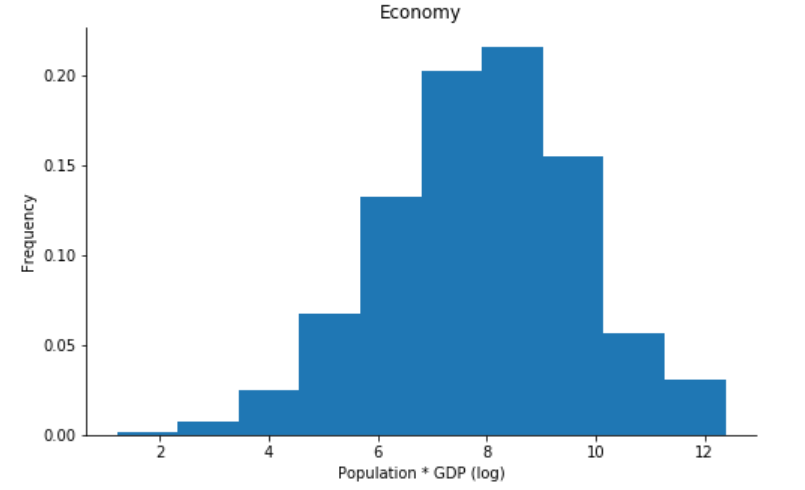Fig. 2. Two histograms showing the distribution of observations before log transformation (left graph), and after (right graph)

# Modeling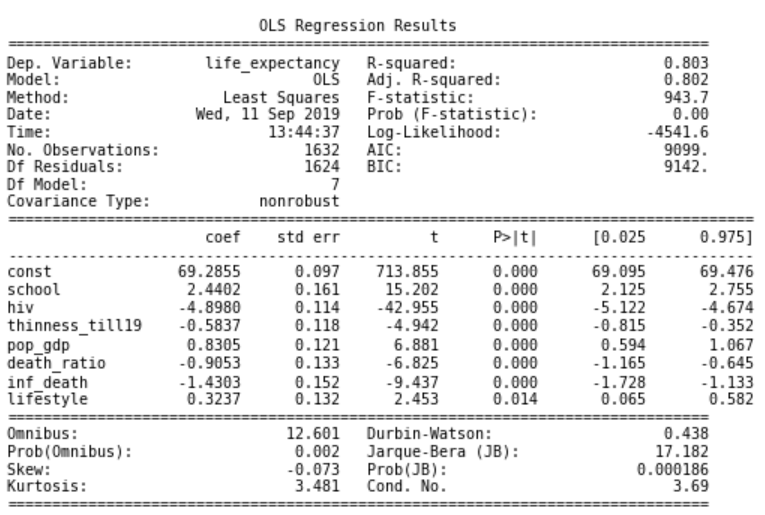Fig. 3. Model summary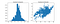Fig. 4. The residual error histogram (left) and residual error scatter plot (right).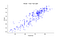Fig. 5. The model’s prediction versus the actual life expectancy. MSE = 3.022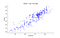Fig. 5 The inclusive of all predictors model predictions versus the actual life expectancy. MSE = 2.995